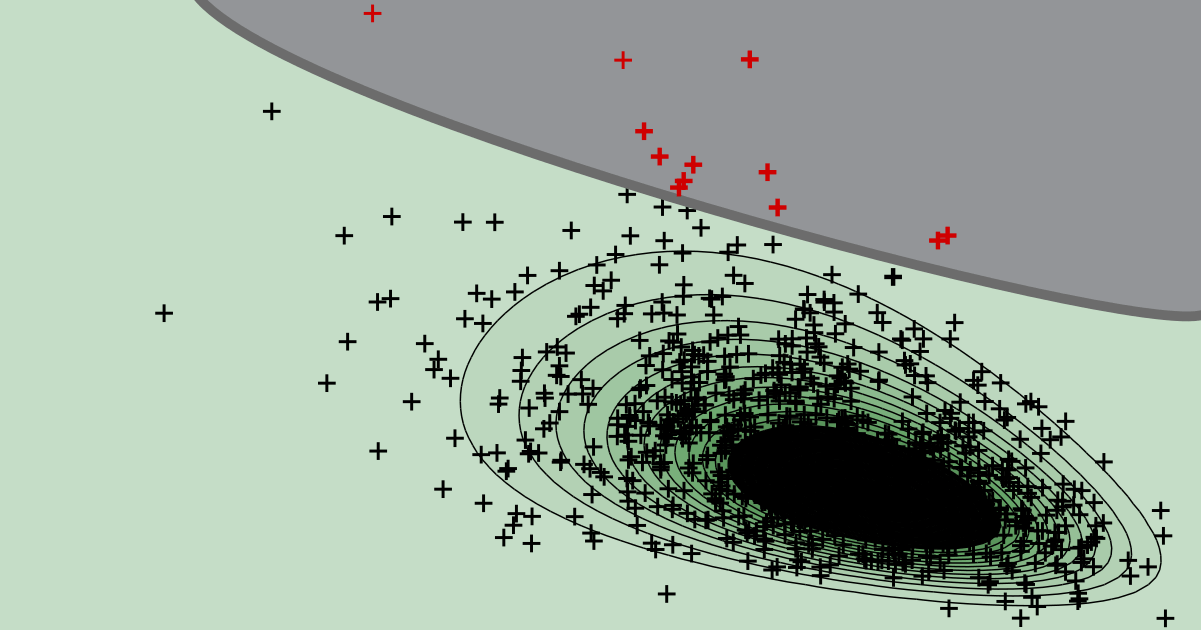# rare event analysis

Reliability analysis

## What is the 'safety margin' in structural reliability analysis?

The difference between the capacity and the demand is the safety margin.

Reliability analysis

## What is the 'basic structural reliability problem'?

When the limit state function can be expressed as the difference between capacity and demand, the problem is sometimes referred to as basic reliability problem.

ONLINE TOOLReliability analysis

## Post-processing of Monte Carlo simulation

Use our web app to quantify the uncertainty about the probability of failure based on a conducted Monte Carlo simulation.
try it ...

Reliability analysis

## Why should you avoid Normal confidence intervals for Monte Carlo simulation?

The underlying distribution can be highly skewed, even if the total number of samples in the Monte Carlo simultion is very large. This is why the Normal approximation often performs poorly in practice.

Reliability analysis

## Why to avoid the coefficient of variation in the context of a Monte Carlo simulation?

The distribution quantifying the uncertainty about the probability of failure can be highly skewed, even for a large number of samples. The coefficient of variation is easier to interprete for symmetric distributions.

STRUREL

## How to employ Monte Carlo simulation in COMREL?

After a brief overview of Monte Carlo simulation (MCS) for structural reliability, we explain the details of conducting a MCS in COMREL.

Reliability analysis

## How to interpret the outcome of a Monte Carlo simulation if no failures occurred?

Even if Monte Carlo simulation returns not a single sample in the failure domain, we can still quantify the uncertainty about whether a specified target reliability level is maintained.

STRUREL

## How to employ a MATLAB limit state function in STRUREL?

Integrating a MATLAB model into a reliability analysis is very straightforward in STRUREL. We explain the basic steps using a simple toy example.

STRUREL

## How to employ a Python limit state function in STRUREL?

Integrating a Python model into a reliability analysis is very straightforward in STRUREL. We explain the basic steps using a simple toy example.